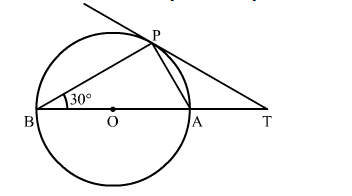# In the given figure, O is the centre of the circle and TP is the tangent to the circle from an external point T.`
Question:

In the given figure, O is the centre of the circle and TP is the tangent to the circle from an external point T. If ∠PBT = 30 , prove that
BA : AT = 2 : 1Solution:

AB is the chord passing through the centre
So, AB is the diameter
Since, angle in a semi circle is a right angle
∴∠APB = 90
By using alternate segment theorem
We have ∠APB = ∠PAT = 30
Now, in △APB
∠BAP + ∠APB + ∠BAP = 180      (Angle sum property of triangle)
⇒ ∠BAP = 180 − 90 − 30 = 60
Now, ∠BAP = ∠APT + ∠PTA        (Exterior angle property)
⇒ 60 = 30 + ∠PTA
⇒ ∠PTA = 60 − 30 = 30
We know that sides opposite to equal angles are equal.
∴ AP = AT
In right triangle ABP

$\sin \angle \mathrm{ABP}=\frac{\mathrm{AP}}{\mathrm{BA}}$

$\Rightarrow \sin 30^{\circ}=\frac{\mathrm{AT}}{\mathrm{BA}}$

$\Rightarrow \frac{1}{2}=\frac{\mathrm{AT}}{\mathrm{BA}}$

$\therefore \mathrm{BA}: \mathrm{AT}=2: 1$